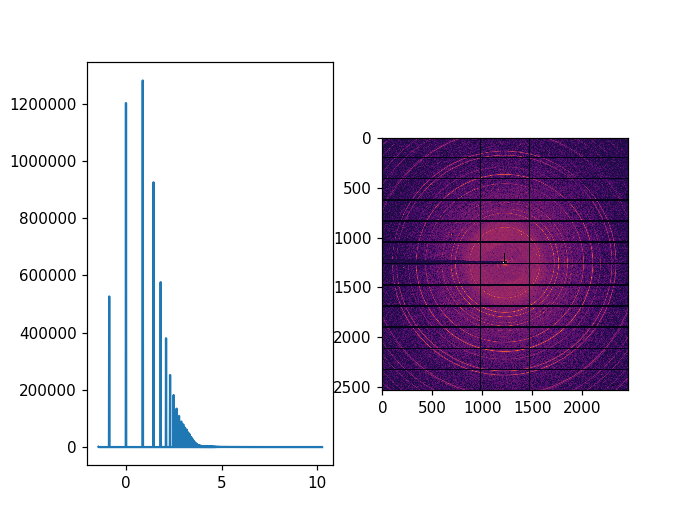# Image processing performance optimisation¶

The idea of this tutorial is to give some suggestion how optimise image processing. In the following example we decompress a CBF image from a Pilatus 6M, take the log-scale and calculate the histogram of pixel intensities before plotting. This very simple operation takes >300ms, what makes it unpractical for live display of images coming from a detector.

:

%pylab nbagg

Populating the interactive namespace from numpy and matplotlib

:

import time, os
start_time = time.time()
import silx
print("Using silx version ",silx.version)
from silx.resources import ExternalResources
print("filename", os.path.basename(fname))
import fabio
nbins = 1000

Using silx version  0.7.0-dev0
filename powder_200_2_0001.cbf

/users/kieffer/VirtualEnvs/py3/lib/python3.5/site-packages/h5py/__init__.py:36: FutureWarning: Conversion of the second argument of issubdtype from float to np.floating is deprecated. In future, it will be treated as np.float64 == np.dtype(float).type.
from ._conv import register_converters as _register_converters

:

%%time

#Display an image and the histogram of values (in log scale)
img = fabio.open(fname).data
log_img = numpy.arcsinh(img) # arcsinh is well behaved log-like function
his, edges = numpy.histogram(log_img, nbins)

CPU times: user 252 ms, sys: 32 ms, total: 284 ms
Wall time: 280 ms

:

fig, ax = subplots(1,2,)
center = (edges[1:] + edges[:-1])/2.0 # this is the center of the bins
ax.imshow(log_img, cmap="inferno")
ax.plot(center,his):

[<matplotlib.lines.Line2D at 0x7fdf03bb7cf8>]


## Optimised GPU code¶

The code below prepares a GPU to be able to perform the same calculation as in the previous example.

3 operations have to be carried out:

• Decompress the CBF image
• Calculate the log scale (arcsinh values)
• Calculate the histogram

Both can be performed on the GPU without additional memory transfer

:

#switch this to "CPU" to have a fail safe
devicetype = "GPU"
from silx.opencl.codec.byte_offset import ByteOffset
from silx.opencl.image import ImageProcessing
import pyopencl, pyopencl.array, pyopencl.elementwise
cbf = fabio.cbfimage.CbfImage()
bo = ByteOffset(os.path.getsize(fname), img.size, devicetype=devicetype)
ash = pyopencl.elementwise.ElementwiseKernel(bo.ctx,
arguments="float* data, float* res",
operation="res[i] = asinh(data[i])",
name='arcsinh_kernel')
ip = ImageProcessing(template=img, ctx=bo.ctx)
res = pyopencl.array.empty(bo.queue, img.shape, dtype=float32)

:

%%time
dec = bo(raw, as_float=True)
ash(dec, res)
his, edges =  ip.histogram(res, nbins, copy=False)
log_img = res.get()

CPU times: user 16 ms, sys: 16 ms, total: 32 ms
Wall time: 28.8 ms

:

fig, ax = subplots(1,2,)
center = (edges[1:] + edges[:-1])/2.0 # this is the center of the bins
ax.imshow(log_img, cmap="inferno")
ax.plot(center,his):

[<matplotlib.lines.Line2D at 0x7fdebd059390>]


The results are the same and the processing time is 6x faster. Hence, one can envisage realtime visualisation of images coming from detectors.

## Investigation of the timings¶

:

ip.set_profiling(True)
bo.set_profiling(True)
ip.reset_log()
bo.reset_log()
dec = bo(raw, as_float=True)
ash(dec, res)
his, edges =  ip.histogram(res, nbins, copy=False)
log_img = res.get()
import os
print(os.linesep.join(bo.log_profile()))
print(os.linesep.join(ip.log_profile()))


Profiling info for OpenCL ByteOffset
copy raw H -> D:  0.972ms
memset counter:     0.005ms
mark exceptions:     0.092ms
copy counter D -> H:  0.002ms
treat_exceptions:     0.027ms
double scan:     0.200ms
copy_results:     0.141ms
________________________________________________________________________________
Total execution time:     1.530ms

Profiling info for OpenCL ImageProcessing
copy D->D :  0.104ms
max_min_stage1:     0.069ms
max_min_stage2:     0.009ms
histogram:     0.098ms
________________________________________________________________________________
Total execution time:     0.281ms


## Conclusion¶

This notebook explains how to optimise some heavy numerical processing up to 10x speed-up for realtime image processing using GPU.

[ ]: